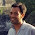## MATLAB

There is a feature in MATLAB called "cheap copies" that makes expanding an array of different objects of the same class a snap. When I say different objects I mean that in the case of handle class objects, each object references a unique object, so that a change in one of its properties affects only it, and is not simultaneously changed in all of the other objects. That's what would happen if all of the objects were really references to the exact same object.

For example assume you have the following MATLAB handle class.
```classdef MyClass < handle
properties
a
end
methods
function obj = MyClass(a)
obj.a = a;
end
end
end
```
Then ...
```myClassArray(3) = MyClass(5)
```
...creates an array of three `MyClass` objects which are unique.

## Python

In Python the way to do this is with `deepcopy` from the builtin `copy` package.

For example assume you have the following Python class.
```from copy import deepcopy

class MyClass(object):
def __init__(self, a=5):
self.a = a

# make 3 copies, but all point to the same instance

myClassArray = [MyClass(a=5)] * 3

# now make deep copies of each object in the array except the first
# one, so that they are now all unique instances

myClassArray[1:] = [deepcopy(dummy) for dummy in myClassArray[1:]]
```
Enjoy! For objects that are computationally expensive, this is a very fast way to build a bunch of identical but unique "copies" of objects of the same class.

#### 1 comment:

1.This example also demonstrates how concise Python code is compared to MATLAB - 3 lines vs. 10 lines!